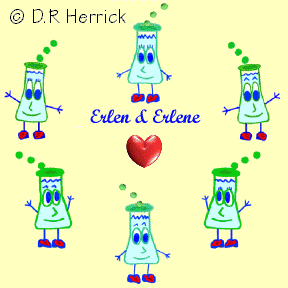Dr. Herrick's CH222 Pressure Quiz 4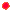Gases and Kinetic-Molecular Theory Copyright D. Herrick20 Questions from past exams. Practice for speed.  Aim for 2 minutes per problem.

 Constants: Avogadro's number: NA = 6.0221 × 1023 / mole Universal gas constant: R =  0.08206 L-atm/mol-K = 8.314 J/mol-K Pressure:  1 atm = 760 mmHg = 760 torr Normal freezing point of water:  0.00ºC = 273.15K Normal boiling point of water:  100.00ºC = 373.15K Triple point of water:  0.01ºC = 273.16K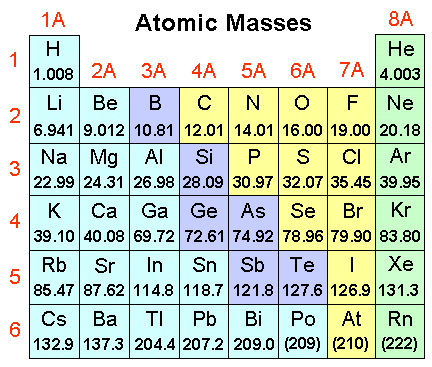1 The initial (V1) and final (V2) volumes of a gas sample satisfy ____ when the absolute temperature is doubled and the pressure is tripled.
 A) V2 = 0.667 V1 D) V2 = 1.00 V1 B) V2 = 1.50 V1 E) V2 = 2.67 V1 C) V2  = 0.38 V1 F) V2 = 6.00 V1
 2 Convert 0.50 atm to mmHg.
 A) 760 B) 1520 C) 380 D) 547 E) 670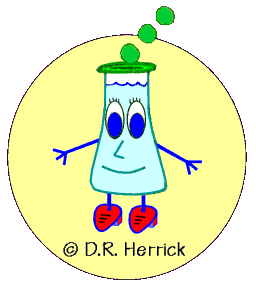3 According to Boyle a gas sample at a fixed temperature satisfies
 A) V/P2 is constant D) V/P is constant B) PV is constant E) V and P are constant C) P/V2 is constant F) PV2 is constant
 4 The initial (V1) and final (V2) volumes of a gas satisfy ______ when the absolute temperature is doubled and the pressure is reduced by 50%.
 A) V2 = 0.25 V1 D) V2  = 4.00 V1 B) V2 = 0.50 V1 E) V2 = 2.00 V1 C) V2 = 1.00 V1 F) V2 = 0.75 V1
 5 The volume of 2 moles of an ideal gas at 200°C and 1500 mmHg is
 A) 27.1 L B) 30.0 L C) 32.6 L D) 44.8 L E) 39.3
 6 A 2.00 L cylinder containing 84.0 g of nitrogen gas can hold pressures up to 100 atm.   What is the highest temperature the gas can be heated before the cylinder explodes?
 A) 683°C B) 420°C C) 540°C D) 722°C E) 492°C
 7 The temperature of a gas in a sealed container changes from 20°C to 40°C.  If the volume remains constant, the pressure (mmHg) will change from 740 mmHg to
 A) 790 B) 812 C) 722 D) 1480 E) 760

 solidliquidgas8 A sample of H2(g) has a volume of 4 L, and a sample of O2(g) at the same temperature and pressure has a volume of 2 L.  Which statement is correct?
 A) The number of  H2  molecules equals the number of  O2  molecules. B) There are twice as many  O2  molecules as H2  molecules. C) There are twice as many H2  molecules as  O2  molecules. D) The mass of the  O2  gas is twice the mass of the H2  gas. E) The mass of the  H2  gas is twice the mass of the O2  gas.
 9 Which gas has the highest density (g/L) at 0.6 atm, 380°C?
 A) C6H6 B)  Xe C)  SO2 D)  Br2 E)  CCl4
 10 A gas mixture of 2 moles of hydrogen and 1 mole of oxygen has a pressure of 810 mmHg.  What is the partial pressure of hydrogen in mmHg?
 A) 810 B) 540 C)  405 D)  270 E)  42011 What mass of phosphorous will react with 52.1 L of O2 gas at STP by         P4(s) + 5 O2(g)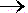P4O10(s)
 A) 57.6 g B) 48.5 g C) 64.1 g D) 50.6 g E) 66.3 g
 12 Analysis of a mixture of  O2  and  N2 gases at 760 torr shows the partial pressure of  O2  is 292 torr.  What is the partial pressure of  N2  in mmHg?
 A) 234 B) 584 C) 468 D) 292 E) 380
 13 Determine the volume relationship when a sample of an ideal gas is taken from T1 = 450K and P1 = 300 mmHg to T2 = 900K and P2 = 900 mmHg.
 A) V2 = 0.33 V1 D) V2 = 1.33 V1 B) V2 = 1.67 V1 E) V2 = 0.75 V1 C) V2 = 1.50 V1 F) V2 = 0.67 V1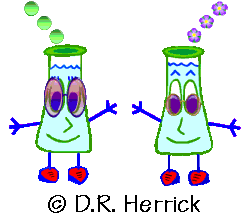14 What volume of oxygen gas at STP will react with 30 L of hydrogen gas at STP?       2 H2(g) + O2(g)2H2O(g)
 A)  30 L B)  33 L C)  0.45 L D)  60 L E) 15 L
 15 A product gas mixture from the reaction     NH4NO3(s)N2O(g) + 2H2O(g) has a pressure of 786 mmHg.  What is the partial pressure (mmHg) of N2O in the mixture?
 A)  128 B) 393 C) 262 D) 480 E) 534
 16 The density (g/L) of all ideal gases at the same temperature and pressure is directly proportional to the _______ of the gas.
 A) molecular weight B) square root of the molecular weight C) reciprocal of the molecular weight. D) reciprocal of the square root of the molecular weight. E) square of the molecular weight.
 17 According to the kinetic theory of gases the absolute temperature of a gas is directly proportional to the ______ of the gas molecules.
 A) average speed B) reciprocal of the average kinetic energy C) square root of the kinetic energy D) average kinetic energy E) most probable speed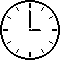18 Properties of a real gas are most likely to deviate from those predicted for an ideal gas when
 A) P is low and T is low B) P is high and T is low C) P is high and T is high D) P is low and T is high
19.

Which distribution for a gas at two different temperatures represents the higher average kinetic energy?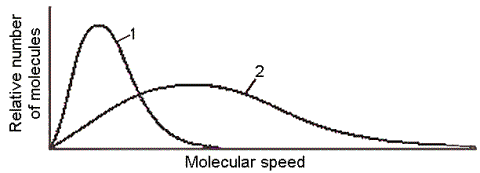A) curve 1 (higher temperature) B) curve 1 (lower temperature) C) curve 2 (higher temperature) D) curve 2 (lower temperature) E) the average kinetic energy is the same for both curves
 20 What do the constants "a" and "b" represent in the van der Waals equation of state for one mole of gas?           (P + a/V2) (V-b) = RT
 A) a = attractive forces between molecules; b = attractive forces between molecules B) a = finite size of molecules; b = attractive forces between molecules C) a = finite size of molecules; b = finite size of molecules D) a = attractive forces between molecules; b = finite size of molecules